# Team:Evry/Programming

Iron coli project

# Programming methods

This part exposes our technical and algorithmical choices, the implementation and the numerical resolution of our models.

## Models implementation

All our models are using the Python language. Python has several major perks:

• Very good floating numbers management
• High level programming
• Object-oriented
• Not an exclusively numerical tool, which allows much more options than Scilab or Matlab

## Numerical resolution of ODEs

We chose not to use the resolution functions included in Scipy to have more coding liberties.
To solve our ODEs, we used a simple Euler method. Here is an example:
Let the following be a Cauchy problem: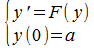Considering the following Taylor expansion: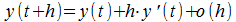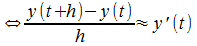Let us define the sequence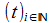such as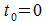and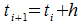And thus, the sequence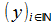such as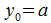and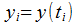Thus, we can define the Euler method: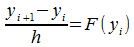Which, standardized, becomes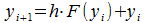.

In the end, all our differential equation systems are implemented like so: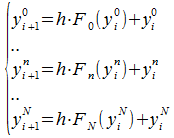where N is the number of equations in the system.

This is how we implemented the equation systems in Python. The fact that we didn't use ODE-solving libraries allowed us to introduce definition domains for some variables, which improved the overall stability in numerical resolutions.
For a simpler and quicker use of repetitive tasks, we also encapsulated everything in a class scheme.